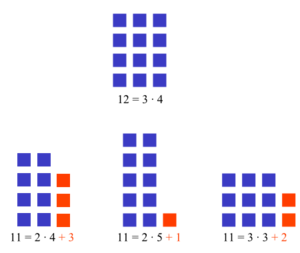# Prime number facts for kids

Kids Encyclopedia FactsHere is another way to think of prime numbers. The number 12 is not prime, because a rectangle can be made, with sides of lengths 4 and 3. This rectangle has an area of 12, because all 12 blocks are used. This cannot be done with 11. No matter how the rectangle is arranged, there will always be blocks left over. 11 must therefore be a prime number

A composite number is a natural number of a particular kind. Any natural number is equal to 1 times itself. If the number is equal to any other numbers multiplied, then the number is a composite number. The smallest composite number is 4, because 2 x 2 = 4. 1 is not a composite number. Every other number is a prime number. The prime numbers are the numbers other than 1 which are not equal to m x n (except 1 x itself). The smallest prime number is 2. There is no largest prime number. The next smallest prime numbers are 3, 5, 7, 11, 13, and so on.

The way that the prime numbers occur is a difficult problem for mathematicians. When a number is larger, it is more difficult to know if it is a prime number. One of the answers is the Prime number theorem. One of the unsolved problems is Goldbach's conjecture.

## How to find small prime numbers

There is a simple method to find a list of prime numbers. Eratosthenes created it. It has the name Sieve of Eratosthenes. It catches numbers that are not prime (like a sieve) and lets the prime numbers pass through.

• On a sheet of paper, write all the whole numbers from 2 up to the number being tested. Do not write down the number 1, because it is not a prime number.
• At the start, all numbers are not crossed out.

The method is always the same:

1. Start with 2. Let p equal 2. Go to the next step.
2. If p is the end of the list, then go to the last step. If p is not the end of the list, go to the next step.
3. Start with p and count out p numbers then cross out that number. Repeat counting off p numbers and crossing out numbers until the end of the list. (This means that all the numbers p x n are crossed out. p x n is a composite number. The first time, this step will cross out 4, 6, 8, and so on. The second time, this step will cross out 3, 6, 9, 12, and so on. 6 and 12 have already been crossed out. Cross them out again. The next time, this will cross out 10, 15, 20, and so on.) Go to the next step.
4. Add 1 to p. Go to the next step.
5. If the new value of p has been crossed out, go back to the last step. (If the new value of p is crossed out, it is composite. Try another value of p)
6. Go back to the 2nd step. (If the new value of p has not been crossed out, then it is a prime number.)
7. (This is the last step). All of the composite numbers are crossed out and all of the prime numbers are not crossed out.

As an example, if this is done up to the number 10, the numbers 2, 3, 5 and 7 are prime numbers, and 4, 6, 8, 9 and 10 are composite numbers.

This method or algorithm takes too long to find very large prime numbers. But it is less complicated than methods used for very large primes, like Fermat's primality test (a primality test is to test whether a number is prime or not) or the Miller-Rabin primality test.

## What prime numbers are used for

Prime numbers are very important in mathematics & computer science. Some real-world uses are given below. Very long prime numbers are hard to solve. It is difficult to find their prime factors, so most of the time, numbers that are probably prime are used for encryption and secret codes.

• Most people have a bank card, where they can get money from their account, using an ATM. This card is protected by a secret access code. Since the code needs to be kept secret, it cannot be stored in cleartext on the card. Encryption is used to store the code in a secret way. This encryption uses multiplications, divisions, and finding remainders of large prime numbers. An algorithm called RSA is often used in practice. It uses the Chinese remainder theorem.
• If someone has a digital signature for their email, encryption is used. This makes sure that no one can fake an email from them. Before signing, a hash value of the message is created. This is then combined with a digital signature to produce a signed message. Methods used are more or less the same as in the first case above.
• Finding the largest prime known so far has become a sport of sorts. Testing if a number is prime can be difficult if the number is large. The largest primes known at any time are usually Mersenne primes because the fastest known test for primality is the Lucas-Lehmer test, which relies on the special form of Mersenne numbers.

## Images for kidsPrime number Facts for Kids. Kiddle Encyclopedia.